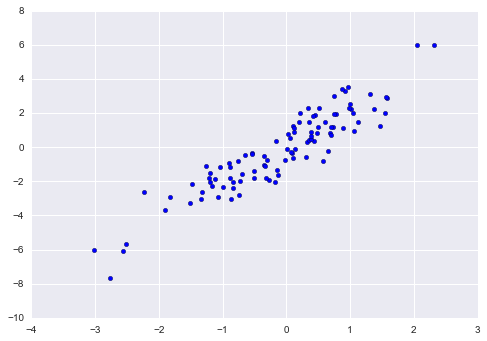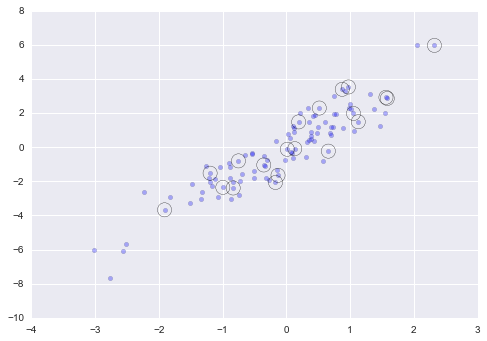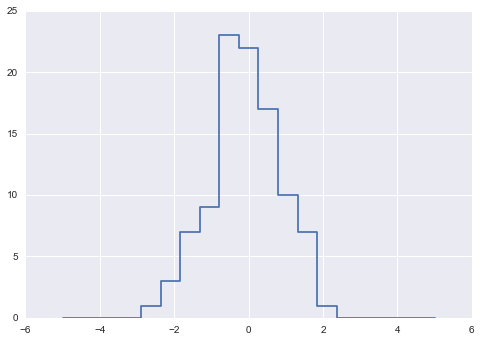## 9.9 花式索引

### 探索花式索引

``````import numpy as np
rand = np.random.RandomState(42)

x = rand.randint(100, size=10)
print(x)

# [51 92 14 71 60 20 82 86 74 74]
``````

``````[x, x, x]

# [71, 86, 14]
``````

``````ind = [3, 7, 4]
x[ind]

# array([71, 86, 60])
``````

``````ind = np.array([[3, 7],
[4, 5]])
x[ind]

'''
array([[71, 86],
[60, 20]])
'''
``````

``````X = np.arange(12).reshape((3, 4))
X

'''
array([[ 0,  1,  2,  3],
[ 4,  5,  6,  7],
[ 8,  9, 10, 11]])
'''
``````

``````row = np.array([0, 1, 2])
col = np.array([2, 1, 3])
X[row, col]

# array([ 2,  5, 11])
``````

``````X[row[:, np.newaxis], col]

'''
array([[ 2,  1,  3],
[ 6,  5,  7],
[10,  9, 11]])
'''
``````

``````row[:, np.newaxis] * col

'''
array([[0, 0, 0],
[2, 1, 3],
[4, 2, 6]])
'''
``````

### 复合索引

``````print(X)

'''
[[ 0  1  2  3]
[ 4  5  6  7]
[ 8  9 10 11]]
'''
``````

``````X[2, [2, 0, 1]]

# array([10,  8,  9])
``````

``````X[1:, [2, 0, 1]]

'''
array([[ 6,  4,  5],
[10,  8,  9]])
'''
``````

``````mask = np.array([1, 0, 1, 0], dtype=bool)

'''
array([[ 0,  2],
[ 4,  6],
[ 8, 10]])
'''
``````

### 示例：选择随机点

``````mean = [0, 0]
cov = [[1, 2],
[2, 5]]
X = rand.multivariate_normal(mean, cov, 100)
X.shape

# (100, 2)
``````

``````%matplotlib inline
import matplotlib.pyplot as plt
import seaborn; seaborn.set()  # 设置绘图风格

plt.scatter(X[:, 0], X[:, 1]);
````````````indices = np.random.choice(X.shape, 20, replace=False)
indices

'''
array([93, 45, 73, 81, 50, 10, 98, 94,  4, 64, 65, 89, 47, 84, 82, 80, 25,
90, 63, 20])
'''

selection = X[indices]  # 花式索引
selection.shape

# (20, 2)
``````

``````plt.scatter(X[:, 0], X[:, 1], alpha=0.3)
plt.scatter(selection[:, 0], selection[:, 1],
facecolor='none', s=200);
``````### 使用花式索引修改值

``````x = np.arange(10)
i = np.array([2, 1, 8, 4])
x[i] = 99
print(x)

# [ 0 99 99  3 99  5  6  7 99  9]
``````

``````x[i] -= 10
print(x)

# [ 0 89 89  3 89  5  6  7 89  9]
``````

``````x = np.zeros(10)
x[[0, 0]] = [4, 6]
print(x)

# [ 6.  0.  0.  0.  0.  0.  0.  0.  0.  0.]
``````

4去了哪里？ 这个操作的结果是首先赋值`x = 4`，然后是`x = 6`。 结果当然是`x`包含值 6。很合理，但考虑这个操作：

``````i = [2, 3, 3, 4, 4, 4]
x[i] += 1
x

# array([ 6.,  0.,  1.,  1.,  1.,  0.,  0.,  0.,  0.,  0.])
``````

``````x = np.zeros(10)
print(x)

# [ 0.  0.  1.  2.  3.  0.  0.  0.  0.  0.]
``````

`at()`方法使用指定的值（此处为 1）在指定的索引处（此处为`i`），执行给定运算符的原地应用。另一种本质上类似的方法是`ufunc``reduceat()`方法，你可以阅读 NumPy 文档。

### 示例：数据分箱

``````np.random.seed(42)
x = np.random.randn(100)

# 手动计算直方图
bins = np.linspace(-5, 5, 20)
counts = np.zeros_like(bins)

# 为每个 x 寻找合适的桶
i = np.searchsorted(bins, x)

# 给每个这些桶加 1
``````

``````# 绘制结果
plt.plot(bins, counts, linestyle='steps');
````````````plt.hist(x, bins, histtype='step');
``````

``````print("NumPy routine:")
%timeit counts, edges = np.histogram(x, bins)

print("Custom routine:")

'''
NumPy routine:
10000 loops, best of 3: 97.6 μs per loop
Custom routine:
10000 loops, best of 3: 19.5 μs per loop
'''
``````

``````x = np.random.randn(1000000)
print("NumPy routine:")
%timeit counts, edges = np.histogram(x, bins)

print("Custom routine:")

'''
NumPy routine:
10 loops, best of 3: 68.7 ms per loop
Custom routine:
10 loops, best of 3: 135 ms per loop
'''
``````